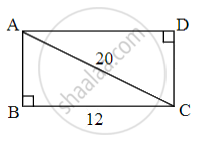# Choose the correct alternative: A rectangle having length of a side is 12 and length of diagonal is 20, then what is length of other side? - Geometry

MCQ

Choose the correct alternative:

A rectangle having length of a side is 12 and length of diagonal is 20, then what is length of other side?

• 2

• 13

• 5

• 16

#### Solution

16Let ABCD be the given rectangle.

AC = 20 units and BC = 12 units

In ∆ABC,

∠B = 90°

∴ AC2 = AB2 + BC2      ...[Pythagoras theorem]

∴ 202 = AB2 + 122

∴ 400 = AB2 + 144

∴ AB2 = 256

∴ AB = 16 units

Concept: Converse of Pythagoras Theorem
Is there an error in this question or solution?

Share